# Electrochemical Lab Manual Equations

• Anson Equation
•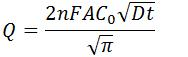• Q is charge (C)
• n is number of electrons transferred
• F is Faraday’s Constant (96,485.3 C/mol)
• A is surface area of the working electrode (cm2)
• C0 is initial concentration of the analyte (mol/cm3)
• D is diffusion coefficient of the analyte (cm2/s)
• t is time (s)

• Corrosion Rate
•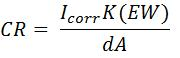• CR is corrosion rate in milli-inches per year (mpy)
• Icorr is corrosion current (A)
• K is constant that defines the units for corrosion (1.288 x 105 milli-inches/A·cm·year)
• EW is equivalent weight (g/equivalent)
• d is density (g/cm3)
• A is area of the electrode (cm2)

• Cottrell Equation
•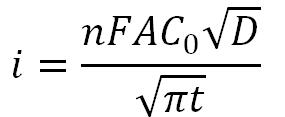• i is current (A)
• n is number of electrons transferred
• F is Faraday’s Constant (96,485.3 C/mol)
• A is surface area of the working electrode (cm2)
• C0 is initial concentration of the analyte (mol/cm3)
• D is diffusion coefficient of the analyte (cm2/s)
• t is time (s)

• Diffusion Layer Thickness
•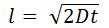• l is distance (cm)
• D is diffusion coefficient of the analyte (cm2/s)
• t is time (s)

•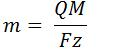• m is mass (g)
• Q is charge (C)
• M is molar mass (g/mol)
• F is Faraday’s Constant (96,485.3 C/mol)
• z is valance number

• Fick’s First Law of Diffusion
•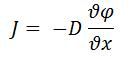• J is diffusional flux (mol/m2s)
• D is diffusion coefficient of the analyte (m2/s)
•is concentration (mol/m3)
• x is position (m)
•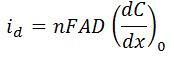• id is diffusion limited current (A)
• n is the number of electrons transferred
• F is Faraday’s Constant (96,485.3 C/mol)
• A is surface area of the working electrode (cm2)
• D is diffusion coefficient of the analyte (cm2/s)
• (dC/dx)0 is concentration gradient at the surface of the electrode

• Formal Peak Potential
•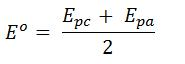• Eo is the formal potential of the analyte
• Epc is the cathodic peak potential
• Epa is the anodic peak potential

• Levich Equation
•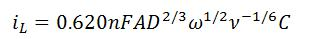• iL is Levich current (A)
• n is the number of electrons transferred
• F is Faraday’s Constant (96,485.3 C/mol)
• A is surface area of the working electrode (cm2)
• D is diffusion coefficient of the analyte (cm2/s)
•is the angular rotation rate of the electrode (rad/s)
• ν is the kinematic viscosity (cm2/s)
• C is concentration of the analyte (mol/cm3)

• Nernst Equation
•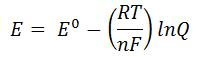•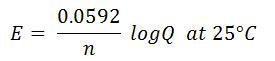• E is cell potential at the temperature of interest
• Eo is the standard cell potential
• R is the Molar Gas Constant (8.31446 J/mol•K)
• T is temperature (K)
• n is number of electrons transferred
• F is Faraday’s Constant (96,485.3 C/mol)
• Q is the reaction quotient

• Ohm’s Law
•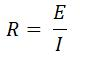• R is resistance
• E is applied potential
• I is applied current

• Peak-to-peak separation for reversible reaction
•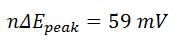• n is the number of electrons transferred
•Epeak is the different between the cathodic and anodic peak potentials

• Randles – Sevcik equation
•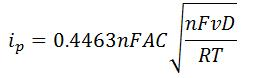•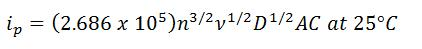• n is the number of electrons transferred
• F is Faraday’s Constant (96,485.3 C/mol)
• A is surface area of the working electrode (cm2)
• D is diffusion coefficient of the analyte (cm2/s)
• R is the Molar Gas Constant (8.31446 J/mol•K)
• T is temperature (K)

• Ratio of peak heights for a reversible reaction
•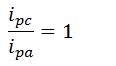• ipc is cathodic peak current
• ipa is anodic peak current

• Steady-State Current at a Microelectrode
•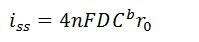• I is steady-state current (A)
• n is the number of electrons transferred
• F is Faraday’s Constant (96,485.3 C/mol)
• D is diffusion coefficient of the analyte (cm2/s)
• Cb is analyte concentration (mol/cm3)
• r0 is radius of the electrode (cm)

• Stern Geary Equation
•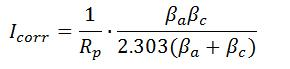• Icorr is corrosion current (A),
• Rp is polarization resistance
• βa is anodic Tafel constant
• βc is cathodic Tafel constant